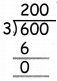Students can download 5th Maths Term 3 Chapter 6 Fractions 6.7 Questions and Answers, Notes, Samacheer Kalvi 5th Maths Guide Pdf helps you to revise the complete Tamilnadu State Board New Syllabus, helps students complete homework assignments and to score high marks in board exams.

Tamilnadu Samacheer Kalvi 5th Maths Solutions Term 3 Chapter 6 Fractions 6.7

Question 1.
Multiply the following
(i) $$\frac{1}{7}$$ × 4
$$\frac{1 \times 4}{7}=\frac{4}{7}$$

(ii) $$\frac{3}{8}$$ × 5
$$\frac{3 \times 5}{8}=\frac{15}{8}$$(iii) $$\frac{7}{11}$$ × 6
$$\frac{7 \times 6}{11}=\frac{42}{11}$$

(iv) $$\frac{21}{50}$$ × 2
$$\frac{21 \times 2}{50}=\frac{42}{50}$$

(v) $$\frac{15}{32}$$ × 3
$$\frac{15 \times 3}{32}=\frac{45}{32}$$John has 300 ml of water in a glass and he drinks $$\frac{2}{3}$$ml of water. How many ml of water does he drink?
$$\frac{2}{3}$$ml of water = $$\frac{2}{3}$$ × 300 = $$\frac{2 \times 300}{3}=\frac{600}{3}$$